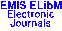## Publications de l'Institut Mathématique (Beograd)Vol. 37(51), 1985 · Contents

 Journal Home All Journals ELibM Home EMIS Home Radomir S. Stankovi\'c: Some remarks on the canonical form for pseudo-Boolean functions Milan Bo\v zi\'c: Semantics for some intermediate logics Miodrag Ra\v skovi\'c: Model theory for $L_{\Cal AM}$ logic Miodrag Ra\v skovi\'c: An application of nonstandard analysis to functional equations \v Zarko Mijajlovi\'c: On a proof of Erd\"s--Monk theorem Ivan Gutman: On some graphic polynomials whose zeros are real Michael G. Voskoglou: Simple skew polynomial rings Nata\v sa Bo\v zovi\'c and Sava Krsti\'c: Algorithmic problems related to the direct prodict of groups Nata\v sa Bo\v zovi\'c: First order classes of groups having no groups with a given property S.J. Pride: G.D. Anderson and M.K. Vamanamurthy: Inequalities for elliptic integrals Suresh P. Singh, Govind Prasad: On approximation by modified Bernstein polynomials N.V. Patel and V.M. Shah: On the absolute summability of lacunary Fourier series Du\v san Georgijevi\'c: Bases from orthogonal subspaces obtained by evaluation of the reproducing kernel Jovan Ke\v cki\'c: The general linear equation on vector spaces J.M. Hernando, E. Reyes and P.M. Gadea: Integrability of tensor structures of electromagnetic type E. \v Sili, B. Jovanovi\'c and L. Ivanovi\'c: On the construction of finite difference schemes approximating generalized solutions B.S. Jovanovi\'c, L.D. Ivanovi\'c and E, \v Sili: O shodimosti raznostnyh shem dlya uravneniya $-\Delta u+cu=f$ na obobwennyh resheniyah iz \$W_{2,*}^s(-\infty Publication date for this issue: 2 Nov 2001. This page was last modified: 16 Nov 2001. © 2001 Mathematical Institute of the Serbian Academy of Science and Arts © 2001 ELibM for the EMIS Electronic Edition